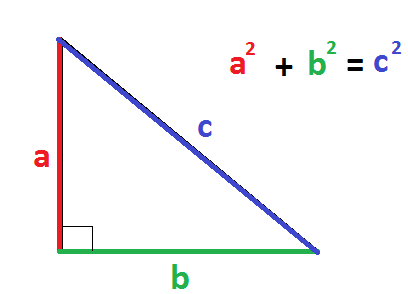# A triangle has sides with lengths of 57 kilometers, 71 kilometers, and 95 kilometers. Is it a right triangle?

Apr 29, 2018

This is NOT a right triangle.

#### Explanation:

To see whether something is a right triangle given the side lengths, we use the Pythagorean Theorem, shown here:If/when we plug them into the formula and both sides are equal, then we know that the triangle is a right triangle.

We have the lengths of the three sides, so we can plug them into the formula:
${57}^{2} + {71}^{2} = {95}^{2}$

$3249 + 5041 = 9025$

$8290 = 9025$